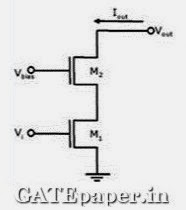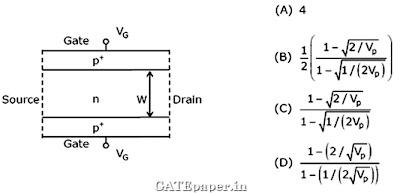### GATE 2008 ECE Video Solutions on EDC (Electronic Devices and Circuits)

1. Which of the following is NOT associated with a PN junction?
a. Junction capacitance
b. Charge storage capacitance
c. Depletion capacitance
d. Channel length modulation

2. Which of the following is TRUE?
a. A silicon wafer heavily doped with boron is a P+ substrate
b. A silicon wafer lightly doped with boron is a P+ substrate
c. A silicon wafer heavily doped with Arsenic is a P+ substrate
d. A silicon wafer lightly doped with Arsenic is a P+ substrate

3. In the following limiter circuit, an input voltage Vi = 10sin100πt applied. Assume that the diode drop is 0.7 volts when it is forward biased. The zener breakdown voltage is 6.8 volts.
The maximum and minimum values of the output voltage respectively area. 6.1 volts, -0.7 volts
b. 0.7 volts, -7.5 volts
c. 7.5 volts, -0.7 volts
d. 7.5 volts, -7.5 volts

4. A silicon wafer has 100nm of oxide on it and is inserted in a furnace at a temperature above 1000oC for further oxidation in dry oxygen. The oxidation rate
a. Is independent of current oxide thickness and temperature
b. Is independent of current oxide thickness but depends on temperature
c. Slows down as the oxide grows
d. Is zero as the existing oxide prevents further oxidation

5. The drain current of a MOSFET in saturation is given by ID = K(VGS-VT)2, where K is a constant. The magnitude of the Transconductance gm is

6. For the circuit shown in the following figure, transistors M1 and M2 are identical NMOS transistors. Assume that M2 is in saturation and the output is unloaded. The IX is related to Ibias as7. The measured Transconductance gm of an NMOS transistor operating in the linear region is plotted against the gate voltage VG at constant drain voltage VD. Which of the following figures represents the expected dependence of gm on VG?8. Two identical NMOS transistors M1 and M2 are connected as shown below. Vbias is chosen so that both transistors are in saturation. The equivalent gm of the pair is defined to be dIout / dVi at constant Vout, isa. The sum of individual gm’s of the transistors
b. The product of individual gm’s of the transistors
c. Nearly equal to the gm of M1
d. Nearly equal to gm1/gm2 of M2

9. Silicon is doped with boron to a concentration of 4x1017atoms/cm3. Assuming the intrinsic carrier concentration of silicon to be 1.5x1010 cm-3 and the value of KT/q to be 25 mV at 300oK. Compared to undoped silicon, the Fermi level of doped silicon
a. Goes down by 0.13 eV
b. Goes up by 0.13 eV
c. Goes down by 0.427 eV
d. Goes up by 0.427 eV

10. The cross section of a JFET is shown in the figure. Let VG be -2 volts and VP be the initial pinch off voltage. If the width W is doubled (with other geometric parameters and doping levels remaining the same), then the ratio between the mutual Transconductance of the initial and the modified JFET is11. Consider the following assertions.
S1: For zener effect to occur, a very abrupt junction is required
S2: For quantum tunneling to occur, a very narrow energy barrier is required.
Which of the following is correct?
a. Only S2 is true
b. Both are true but S2 is not a reason for S1
c. Both are true but S2 is a reason for S1
d. Both are false